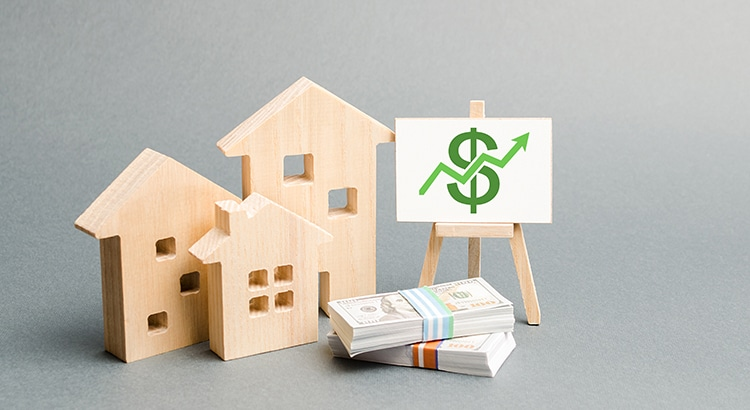March 24, 2023

# Property Appreciation Calculator: How to Calculate Appreciation in Real Estate## Appreciation Calculator

Real estate, stocks, and crypto are what are called appreciating assets — these assets are bought in the hopes that their value increases over time. But not all assets appreciate — let’s take a car, for example. Cars have a limited life and are worth less the minute they’re driven off the lot compared to when they were purchased. Cars are an example of a depreciating asset — its value decreases over time until, eventually, it is no longer usable and becomes worthless.

Investors are, of course, interested in the first kind of asset — appreciating assets. Of all appreciating assets, real estate is not only the most common, but also the most accessible asset for the average investor.

## What is appreciation in real estate?

Natural appreciation can happen in two ways: (1) a tight rental market increasing rents, or (2) a tight real estate market increasing home prices. Either way, natural appreciation happens gradually.

Just as a rising tide lifts all ships, so too does a more favorable market, which is precisely why natural appreciation is also called market appreciation. As long as they maintain their properties, most buy-and-hold real estate investors interested in long-term appreciation see both the value of their homes and the rents generated by their homes increase. There are, of course, fluctuations and cycles in the real estate market - even still, single-family homes have increased in price by almost 67% between the years of 2009 and 2022. In the same period, rent prices have consistently outpaced inflation, leading to increases in cap rates across the long-term rental market.

The second type of appreciation is forced appreciation. Unlike natural appreciation - which is passive and requires little more than maintenance on part of the investor - forced appreciation is active and requires much more. Forced appreciation occurs when a real estate investor proactively takes measures to increase the value of an investment property. This can be achieved by increasing rental income, increasing property value, or some combination of both.

• To increase rental income, an investor could, for example, add two bathrooms to a property and make a 3-bed / 1-bath into a 3-bed / 3-bath property. They could also take a two-bedroom apartment and split it into two, 1-bedroom apartments.
• To increase property value, an investor could purchase a run down property and completely renovate it. Post-renovation, the property’s value would have appreciated (hopefully by more than the cost of the renovation).

Regardless of the method - whether it be natural or forced - both types of appreciation have the same end result: an asset that’ll be worth more tomorrow than it is today.

## How do you calculate real estate appreciation?

The most straightforward way to calculate appreciation is to determine the percentage by which an asset’s value has increased. To do this, all we need to know is an asset’s starting price and ending price. With these two data points, we can calculate the percentage increase in the asset’s price using the following formula.

Appreciation

=

Ending Price - Starting Price

Ending Price

=

% increase of an asset's price

Let’s demonstrate with an example. Say you buy a home for \$100,000 in 2017 and, in 2022, the same home is valued at \$200,000. Using our formula above, we can see that in those five years the value of the house increased by 100%. In other words, the value of this asset doubled.

Appreciation

=

\$200K - \$100K

\$100K

=

\$100K

\$100K

=

1

=

100%

The one thing this method doesn’t take into account is time. Looking at just the percentage by which an asset’s price increased tells you nothing about the duration of the investment, so we have no clue how long it took for the appreciation to occur. And duration matters. Say we have two assets that double -  one is the aforementioned house, which doubles in five years and the other is a remote parcel of land, which doubles in 50 years. Intuitively, we know the better investment is the home that doubles in five years. To show this mathematically, we must calculate each asset’s appreciation rate.

This rate - which is also called a CAGR, or compound annual growth rate - is the annual rate at which an asset would have to grow to reach its terminal price. Appreciation rates are calculated using the formula below.

For the sake of example, let’s calculate the appreciation rates, or CAGRs, for the two investments that both doubled, albeit over different time periods.

These two investments - though they both doubled in value and have appreciated by the exact same amount - have very different rates of appreciation. The 5-year investment has a CAGR that’s nearly 10x the 50-year investment, making it by far the superior investment. It is this exact nuance that makes the rate of appreciation a far more important and telling metric than appreciation in and of itself.

## The importance of appreciation

Understanding appreciation allows you to estimate the upside potential of an investment property. Then, homing in on the rate of this appreciation — whether it be based on historicals of forecasts — allows you to understand the time period necessary for this appreciation to be actualized.

Regardless of whether an investor opts for the more active, forced appreciation strategy or the more passive, natural appreciation one, all appreciation is good appreciation. It is the cornerstone of all real estate investors’ wealth and portfolio-building strategies. At the most basic level, the “goodness” of an investor and their investments is the ability to identify and acquire the most appreciative assets available.